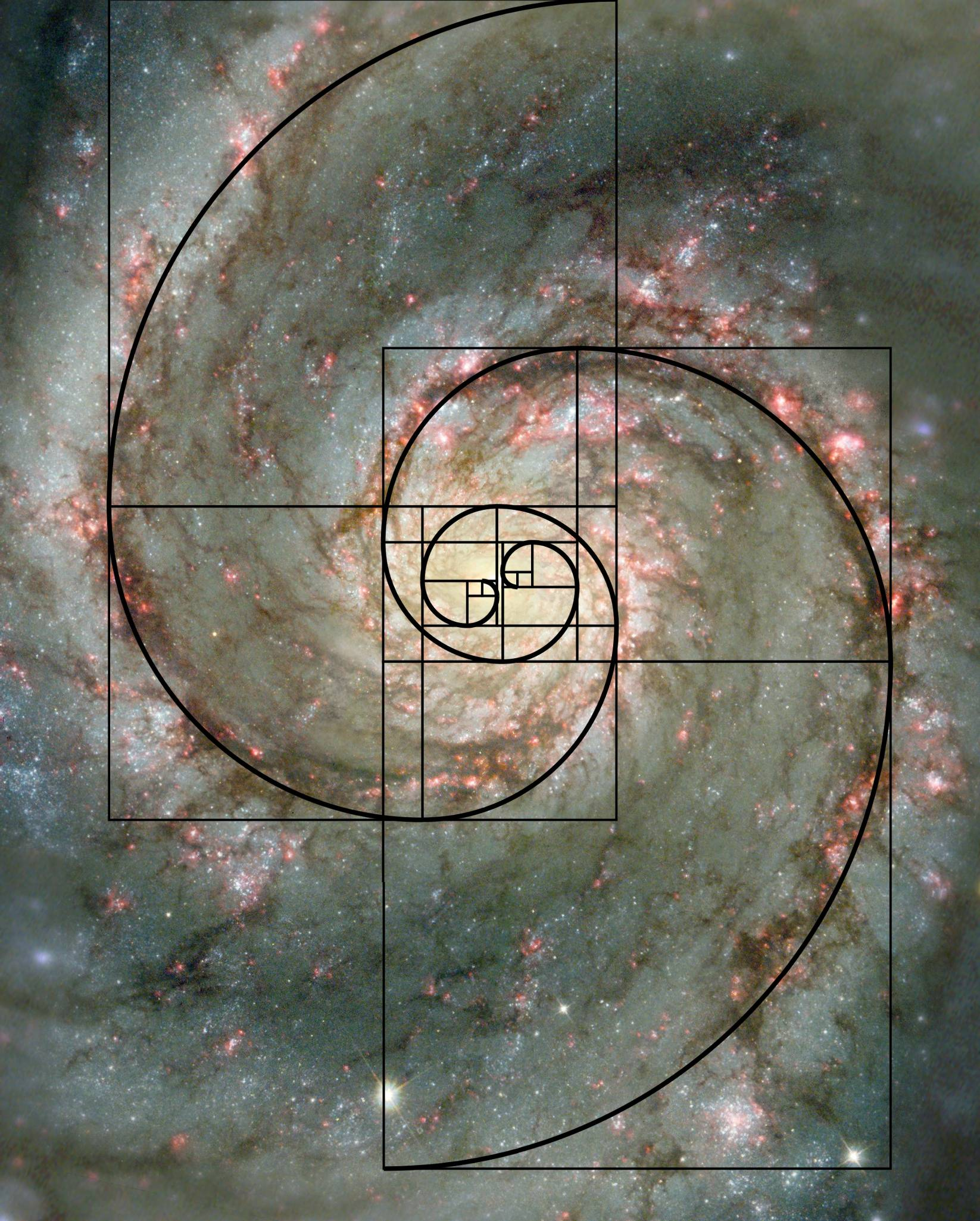# A Golden Fraction I$\frac{100,000,000,000,000,000,000}{99,999,999,989,999,999,999}$

What is the $250^{\text{th}}$ digit to the right of the decimal place of the fraction above?

As an explicit example, the $3^\text{rd}$ digit to the right of the decimal place of 11235.123456789 is 3.

×